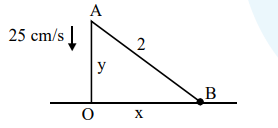# A 2 m ladder leans against a vertical wall.`
Question:

A 2 m ladder leans against a vertical wall. If the top of the ladder begins to slide down the wall at the rate $25 \mathrm{~cm} / \mathrm{sec}$., then the rate (in $\mathrm{cm} / \mathrm{sec}$.) at which the bottom of the ladder slides away from the wall on the horizontal ground when the top of the ladder is $1 \mathrm{~m}$ above the ground is :

1. $25 \sqrt{3}$

2. 25

3. $\frac{25}{\sqrt{3}}$

4. $\frac{25}{3}$

Correct Option: , 3

Solution:$x^{2}+y^{2}=4\left(\frac{d y}{d t}=-25\right)$

$x \frac{d x}{d t}+y \frac{d y}{d t}=0$

$\sqrt{3} \frac{d x}{d t}-1(25)=0$

$\frac{\mathrm{dx}}{\mathrm{dt}}=\frac{25}{\sqrt{3}} \mathrm{~cm} / \mathrm{sec}$